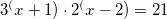PSLE marks the graduation of Primary school students and their entry into Secondary schools as teenagers. Discuss all issues about Secondary schooling here.
questionable_i
BrownBeltPosts: 535
Joined: Sat Oct 10,
Total Likes:1

Can anyone help me?in advance.

Q1) A function f is defined by f(x)=|x^2-3x|. Find the values of x for which f(x)=x-3.

Q2) Find the x-coordinates of the points of intersection of the curve y=|16-x^2| and the line y=33.

Q3) Find the x-coordinates of all the points at which the graph of y=|x^2-9|-5 meets the x-axis.

kennethTutor
OrangeBeltPosts: 39
Joined: Mon Jul 13,
Total Likes:2

KSP2013777 wrote:Thank you, Kenneth Tutor.
Can you help me with another question, Please?Thank you.
The fraction is an improper fraction, since the degree of the numerator is 4 while the degree of the denominator is 3.

Perform long division first. You obtain x + (9 - 3x^2)/(x^3 + 3x)

Finally perform partial fraction decomposition on (9 - 3x^2)/(x^3 + 3x)

Final ans should be: x + 3/x - 6x/(x^2 + 3)

kennethTutor
OrangeBeltPosts: 39
Joined: Mon Jul 13,
Total Likes:2

questionable_i wrote:Can anyone help me?in advance.

Q1) A function f is defined by f(x)=|x^2-3x|. Find the values of x for which f(x)=x-3.

Q2) Find the x-coordinates of the points of intersection of the curve y=|16-x^2| and the line y=33.

Q3) Find the x-coordinates of all the points at which the graph of y=|x^2-9|-5 meets the x-axis.
For Q1, first we sub one equation into another.

|x^2 - 3x| = x - 3

For modulus, there is the positive and negative side.

For the positive (and removing the modulus),

x^2 - 3x = x - 3
x^2 - 4x + 3 = 0
(x - 3)(x - 1) = 0

x = 3 or x = 1

For the negative,

x^2 - 3x = -(x - 3)
x^2 - 3x = -x + 3
x^2 - 2x - 3 = 0
(x - 3)(x + 1) = 0

x = 3 or x = -1

So the values of x are: -1, 1 or 3

You can use the same approach for Q2 and Q3. For Q3, the equation of x-axis is y = 0.

KSP2013777
OrangeBeltPosts: 92
Joined: Fri Dec 28,

Please, can somebody help me solve this question?Is it tangent to curve means Discriminant =0? But how to use Discriminant if there is ln x? Please help. Thank you

PiggyLalala
KiasuGrandMasterPosts: 4053
Joined: Tue May 24,
Total Likes:23

KSP2013777 wrote:Please, can somebody help me solve this question?Is it tangent to curve means Discriminant =0? But how to use Discriminant if there is ln x? Please help. Thank you

this question is not about discriminant = 0. Always remember, discriminant is only for quadratic equation. This is a differentiation question.

So differentiatate and set dy/dx = gradient of the tangent. Find x. Then the y coordinate. Lastly the value of k. Give it a try. 加油。。KSP2013777
OrangeBeltPosts: 92
Joined: Fri Dec 28,

Thank you, piggy lalala. Can get my answer now.KSP2013777
OrangeBeltPosts: 92
Joined: Fri Dec 28,Thank you.

questionable_i
BrownBeltPosts: 535
Joined: Sat Oct 10,
Total Likes:1

Can anyone help me with this question? Thank you.

1) By expressing each of the following equations in the form a^x=b where a and b are real numbers, take logarithms to solve for x.

(a) 2^(x+1)=3^x

(b)PiggyLalala
KiasuGrandMasterPosts: 4053
Joined: Tue May 24,
Total Likes:23Thank you.PiggyLalala
KiasuGrandMasterPosts: 4053
Joined: Tue May 24,
Total Likes:23

(b)